Toggle Accessibility Tools

# 8-7 It's in the System - Concepts and Explanation

## Solving Linear Equations

Students have used tables or graphs to find solutions. They can solve simple linear equations, y = mx + b or mx + b = nx + c, and simple equations with parentheses, y = a(x + b). In this Unit, students solve equations for different variables symbolically, writing equivalent forms of the equation.

### Example

1. Subtract 12x from each side of the equation.

12x + 3y = 9
3y = -12x + 9

2. Divide each side of the equation by 3.

y = -4x + 3

1. Divide each side of the equation by 3.

12x + 3y = 9

Subtract 4x from each side of the equation

4x + y = 3
y = 3 -4x

3. Rearrange the order of terms

y = -4x + 3

## Solving Linear Inequalities

Solving an inequality is very similar to solving a linear equation. The rules for operations with inequalities are identical to those for equations, with one exception. When multiplying (or dividing) an inequality by a negative number, you must reverse the direction of the inequality sign.

### Example

5+ 7 ≤ 42
5x ≤ 35
x ≤ 7

Solving this inequality is similar to solving 5x + 7 = 42. The operations (+, -, x, ÷) are applied to each side of the inequality. You usually show this solution on a number line.-5x + 7 ≤ 42
-5x ≤ 35
x ≥ -7

Reverse the direction of the inequality sign.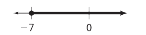## Solving Systems of Linear Equations

There are three standard methods for solving a system of linear equations.

The graphing method involves producing straight-line graphs for each equation and then reading coordinates of intersection points as the solution(s).

The linear combination method relies on two basic principles; (1) If one of the equations is replaced by a new equation formed by adding the two original equations, the solution is unchanged. (2) The solutions of any linear equation Ax + By = C are the same as the solutions of KAx + KBy = KC, where K is a nonzero number.

The equivalent form method is the process of rewriting the equations in y = ax + b form and then setting the two expressions for y equal to each other.

### Example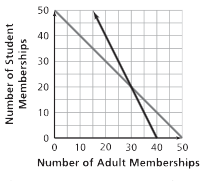The intersection point has coordinates (30, 20), so the solution of the system is x = 30 and y = 20.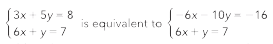Adding the two equations gives -9y = -9. The solution is y = 1 and x = 1.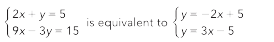Since y = y, -2x + 5 = 3x - 5. The solution is x = 2 and y = 1.

## Solving Systems of Linear Inequalities

Systems of inequalities tend to have infinite solution sets. The solution of a system of distinct, nondisjoint linear inequalities is the intersection of two half-planes, which contain infinitely many points

### Example

In general, there are four regions suggested by a system of linear inequalities such as the following: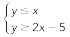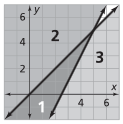Region 1 contains the solutions to the system. Points in Regions 2 and 3 satisfy one, but not both, of the inequalities. The fourth region satisfies neither inequality.